Author: Oscar Cronquist Article last updated on May 11, 2022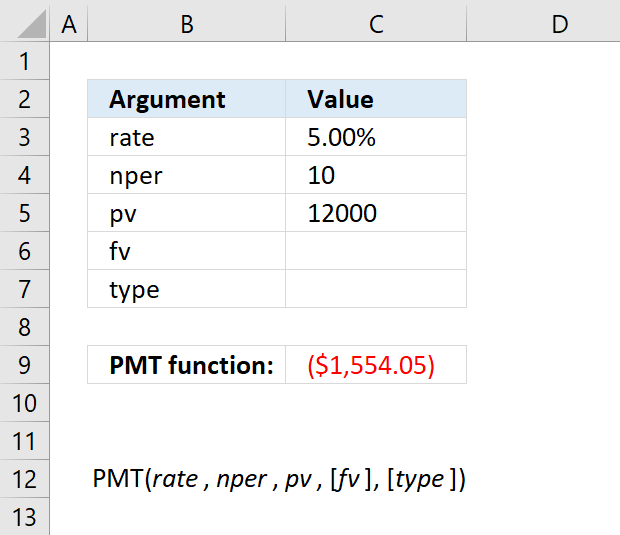The PMT function returns the payment amount needed for borrowing a fixed sum of money based on constant payments and interest rate.

You can also use the PMT function to calculate the amount to save each period to reach a given sum, based on an interest rate and the number of periods.

The above image shows the annual payment needed for a 10-year 12 000 loan based on a 5 percent interest rate.

Formula in cell C7:

=PMT(C3,C4,C5)

### Excel Function Syntax

PMT(rate, nper, pv, [fv], [type])

### Arguments

 Rate Required. The interest rate. nper Required. The number of payments. pv Required. The present value. [fv] Optional. The future value. Default value is 0 (zero). [type] Optional. When payments are due, default value is 0 (zero). 0 (zero) :  at the end of the period. 1 : at the beginning of the period.

Think carefully about what period you use (years, quarterly, months, weekly, days or whatever unit) before you specify the number of payments (nper) and the interest rate.

Monthly payments: For a ten-year loan at an annual interest rate of 5 percent use 5%/12 (rate) and 10*12 (nper).

Annual payments: For a ten-year loan at an annual interest rate of 5 percent use 5% (rate) and 10 (nper).

## 1. Excel Loan Calculator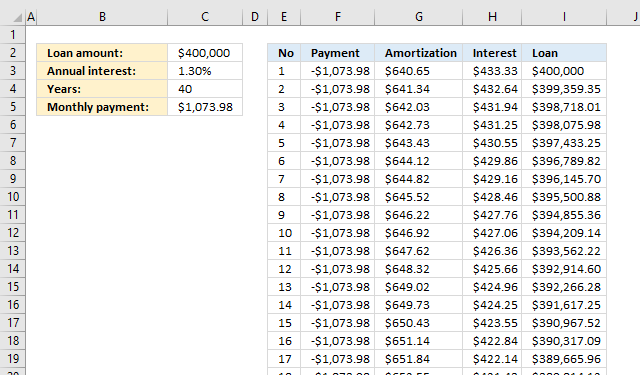The image above describes a loan calculator that displays payment, amortization or principal, interest, and remaining loan per month. The formulas in column E to I resize appropriately based on the values you enter in C2:C4.

 Loan amount This is the total amount you borrow, this can be, for example, a mortgage. Annual interest A percentage that you will pay for the loan annually. Years For how long the loan will be paid. Monthly payment This cell is calculated by Excel using the PMT function, don't enter a value here.

### 1.1 Calculate the monthly payment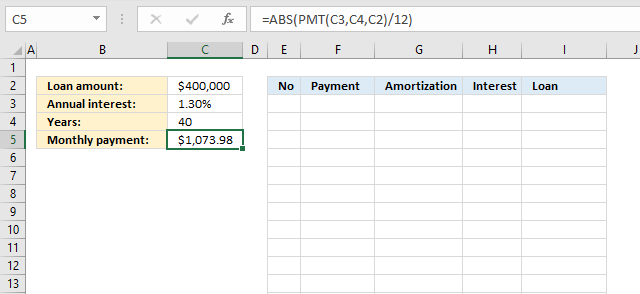Formula in cell C5:

=ABS(PMT(C3, C4, C2)/12)

### Explaining formula in cell E4

#### Step 1 - Calculate yearly payment

The PMT function has the following arguments: PMT(rate, nper, pv, [fv], [type])

rate - interest rate

nper - number of payments

pv - present value

The interest rate is annual. The number of payments is once per year for 40 years. The present value is 400000.

PMT(C3,C4,C2)

becomes

PMT(0.013, 40, 400000)

and returns -12887.7516044148.

#### Step 2 - Calculate monthly payment

Divide yearly payment with 12 to calculate the monthly payment.

PMT(C3,C4,C2)/12

becomes

-12887.7516044148/12

and returns -1073.9793003679.

#### Step 3 - Remove sign

The PMT function returns a negative value meaning the amount will be subtracted from your account. The ABS function removes the sign, we don't need the negative sign in our calculations.

ABS(PMT(C3,C4,C2)/12)

becomes

ABS(-1073.9793003679)

and returns 1073.9793003679.

### 1.2 Create a series of numbers representing months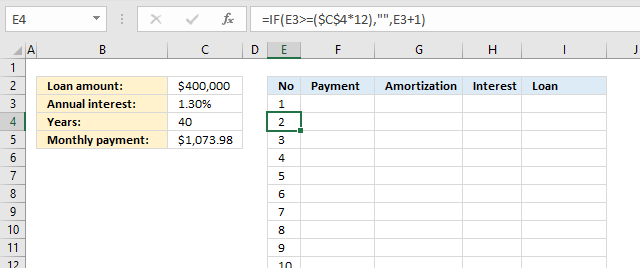Value in cell E3:

1

Formula in cell E4:

=IF(E3>=(\$C\$4*12),"",E3+1)

Copy cell E4 and paste to cells below as far as needed.

### Explaining formula in cell E4

#### Step 1 - Calculate months needed to pay back loan

\$C\$4*12

becomes

40*12 equals 480.

#### Step 2 - Check if the cell above is equal or larger than the number of months

The parentheses allow us to control the order of operation. We want to calculate \$C\$4*12 before we evaluate if the value in cell E3 is larger than or equal to.

E3>=(\$C\$4*12)

becomes

E3>=480

becomes

1>=480

and returns FALSE.

#### Step 3 - Evaluate IF function

The IF function returns one value if the logical test is TRUE and another value if the logical test is FALSE.

IF(logical_test, [value_if_true], [value_if_false])

The IF function allows us to add 1 to cell E3 if E3 is smaller than or equal to the number of payments needed.

IF(E3>=(\$C\$4*12),"",E3+1)

becomes

IF(FALSE,"",E3+1)

becomes

IF(FALSE,"",1+1)

and returns 2 in cell E4.

### 1.3 Populate column F with payments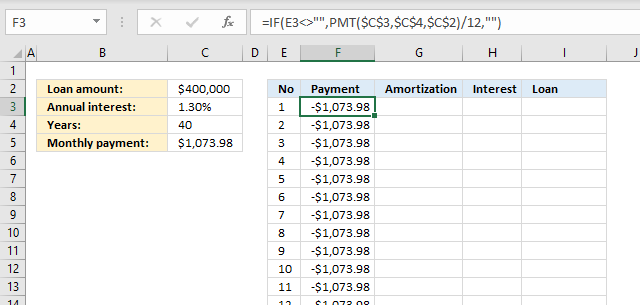Formula in cell F3:

=IF(E3<>"",PMT(\$C\$3,\$C\$4,\$C\$2)/12,"")

### Explaining formula in cell F3

#### Step 1 - Calculate monthly payment

The cell references below are absolute cell references, they don't change when we copy the cell and paste to cells below.

PMT(\$C\$3,\$C\$4,\$C\$2)

becomes

PMT(0.013, 40, 400000)

and returns -12887.7516044148.

#### Step 2 - Divide with 12

PMT(\$C\$3,\$C\$4,\$C\$2)/12

becomes

-12887.7516044148/12

and returns -1073.9793003679

#### Step 3 - Check if adjacent cell is not empty

The IF function makes sure that the adjacent cell in column E is not empty, we don't want to continue calculating when there are no more months.

IF(E3<>"",PMT(\$C\$3,\$C\$4,\$C\$2)/12,"")

becomes

IF(1<>"",PMT(\$C\$3,\$C\$4,\$C\$2)/12,"")

becomes

IF(TRUE,PMT(\$C\$3,\$C\$4,\$C\$2)/12,"")

becomes

IF(TRUE,-1073.9793003679,"")

and returns -1073.9793003679.

### 1.4 Calculate amortization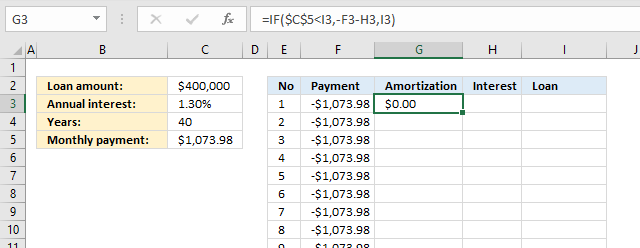The formula in cell G3 returns 0 (zero) now but will return a number when the formulas in cell H3 and I 3 are populated.

Formula in cell G3:

=IF(\$C\$5<I3,-F3-H3,I3)

### Explaining formula in cell G3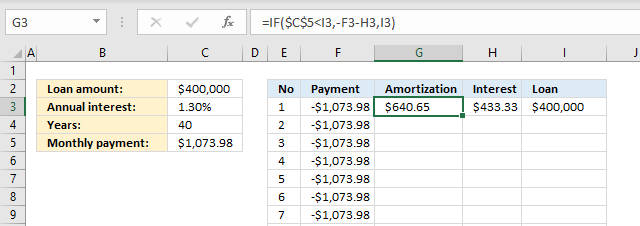Formulas in cell H3 and I3 are explained in the next section. They are populated now in order to explain the calculation in cell G3.

#### Step 1 - Check if remaining loan is larger than monthly payment

The larger than character checks if cell I3 is larger than cell C5. Note that the dollar signs are important here. They make the cell reference to cell C5 absolute meaning it does not change when we copy the cell and paste to cells below.

\$C\$5<I3

becomes

1073.9793003679<400000

and returns TRUE.

#### Step 2 - Calculate amortization

The IF function returns one value if the logical test is TRUE and another value if the logical test is FALSE.

IF(logical_test, [value_if_true], [value_if_false])

IF(\$C\$5<I3,-F3-H3,I3)

becomes

IF(TRUE,-F3-H3,I3)

becomes

IF(TRUE,-1073.9793003679-433.333333333333,I3)

and returns 640.645967034566

### 1.5 Calculate interest per month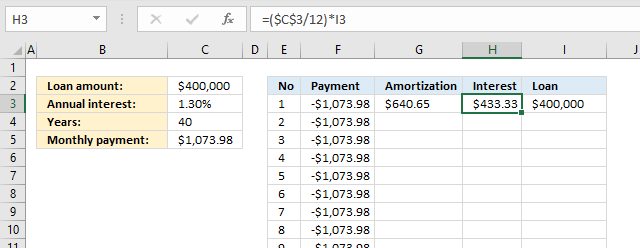Formula in cell H3:

=\$C\$3/12*I3

### Explaining formula in cell H3

#### Step 1 - Divide annual interest with 12

There a re 12 months in a year, to get the monthly interest we divide the annual interest with 12.

\$C\$3/12

becomes

0.013/12

and returns 0.00108333333333333.

#### Step 2 - Multiply with loan

\$C\$3/12*I3

becomes

0.00108333333333333*I3

becomes

0.00108333333333333*400000

and returns 433.333333333333.

### 1.6 Calculate remaining loan per month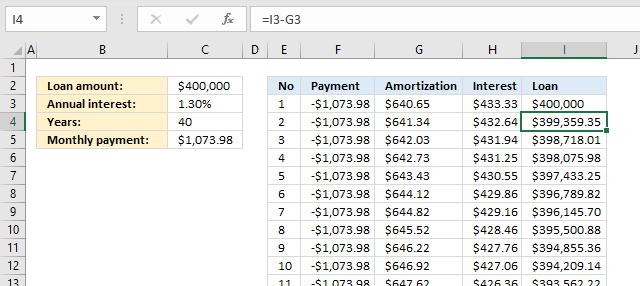Formula in cell I3:

=C2

Formula in cell I4:

=I3-G3

The formula in cell I4 takes the loan in cell I3 and subtracts with the amortization in cell G3.

## 2. Loan calculator - extra payment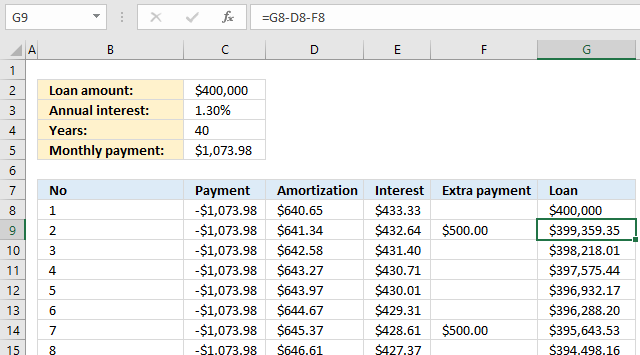The loan schedule shown in the image above allows you to do extra payments, type them in column F and the formula in column G takes care of the calculations.

The only formula that changes compared to the loan calculator above is the formula in column G.

Formula in cell G9:

=G8-D8-F8

The formula in cell G9 takes the loan amount in cell G8 and subtracts with the amortization in cell D8 and the extra payment in cell F8.

Copy cell G9 and paste to cells below as far as needed. The other formulas are explained here: Loan schedule calculator

## 3. Calculate running total interest paid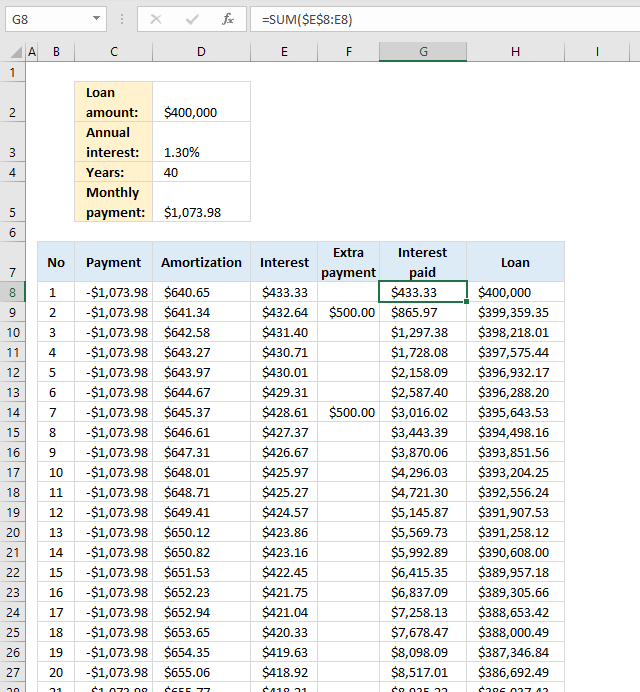The image above demonstrates a formula in column G that displays the sum of interest paid, in other words, a running total of interest paid.

Formula in cell G8:

=SUM(\$E\$8:E8)

### Explaining formula in cell G8

#### Step 1 - Cell reference

Combine an absolute and relative cell reference and you get an expanding cell reference. It grows when you copy the cell and paste to cells below as far as needed.

\$E\$8:E8 returns 433.33.

#### Step 2 - Sum values

The SUM function adds numbers from a cell range and returns a total.

SUM(\$E\$8:E8)

becomes

SUM(433.33)

and returns 433.33 in cell G8.

## 4. Calculate running total principal paid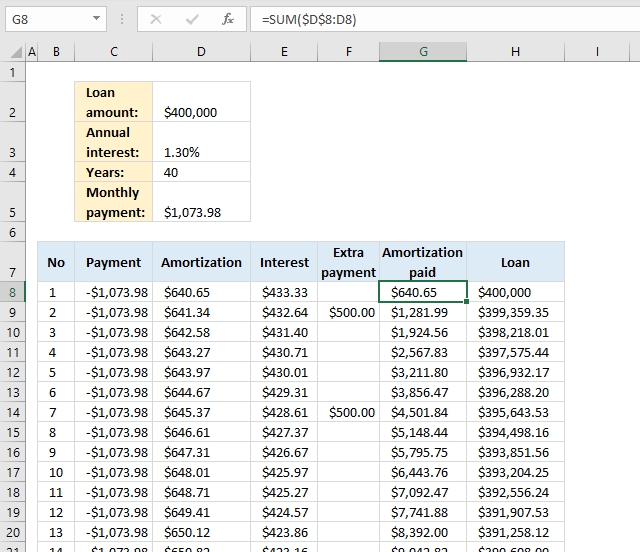The image above demonstrates a formula in column G that displays the sum of principal paid, in other words, a running total of principal paid.

Formula in cell G8:

=SUM(\$D\$8:D8)

Use the following formula in cell G8 if you want to include extra payments to amortization total:

=SUM(\$D\$8:D8)+F8

Check formula explanation here: Calculate running total interest paid

## 5. Calculate the interest saved by making extra payments?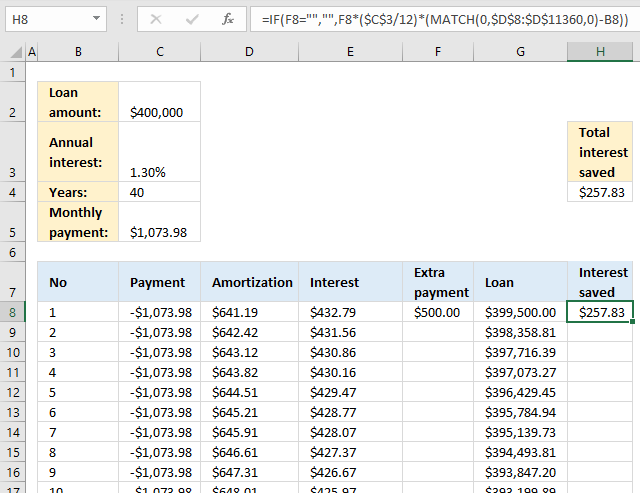The formula in cell H8 calculates the saved interest based on the amount of the extra payment specified in cell F8.

Formula in cell H8:

=IF(F8="","",F8*(\$C\$3/12)*(MATCH(0,\$D\$8:\$D\$11360,0)-B8))

### Explaining formula in cell H8

#### Step 1 - Calculate interest per month

The value in cell C3 is the annual interest. Divide with 12 to get the monthly interest. \$C\$3 is an absolute cell reference, it stays the same when we copy the cell and paste it to cells below.

\$C\$3/12

becomes

0.013/12 equals 0.00108333333333333.

#### Step 2 - Multiply with amount

F8*(\$C\$3/12)

becomes

F8*0.00108333333333333

becomes

500*0.00108333333333333

and returns 0.541666666666667.

#### Step 3 - Find first amortization that is zero

The MATCH function returns the relative position of a given value in an array or cell range.

MATCH(0,\$D\$8:\$D\$11360,0)

returns 477.

#### Step 4 - Subtract current month with last month

(MATCH(0,\$D\$8:\$D\$11360,0)-B8)

becomes

477 - 1 equals 476.

#### Step 5 - Multiply values

F8*(\$C\$3/12)*(MATCH(0,\$D\$8:\$D\$11360,0)-B8)

becomes

0.541666666666667*476

and returns 257.833333333333.

#### Step 6 - Check if cell F8 is empty

IF(F8="","",F8*(\$C\$3/12)*(MATCH(0,\$D\$8:\$D\$11360,0)-B8))

becomes

IF(FALSE,"",F8*(\$C\$3/12)*(MATCH(0,\$D\$8:\$D\$11360,0)-B8))

becomes

IF(FALSE,"",257.833333333333)

and returns 257.833333333333.

## 6. Calculate the months saved by making extra payments?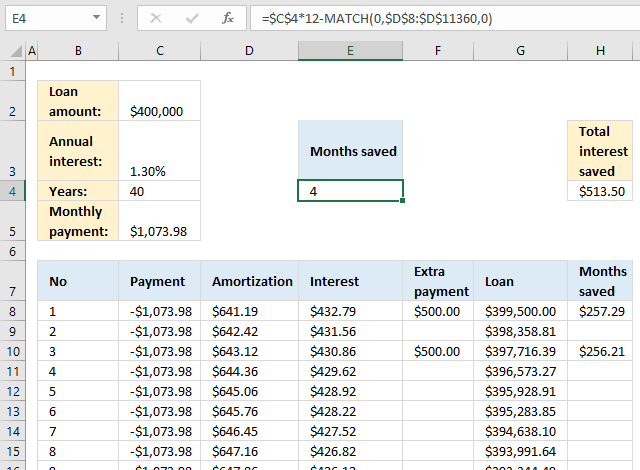The formula in cell E4 calculates the saved months based on the amounts of the extra payments specified in column F.

Formula in cell H8:

=\$C\$4*12-MATCH(0,\$D\$8:\$D\$11360,0)

### Explaining formula in cell H8

#### Step 1 - Calculate total months

\$C\$4*12

becomes

40*12 equals 480.

#### Step 2 - Calculate months needed to repay the loan

The MATCH function returns the relative position of a given value in an array or cell range.

The MATCH function finds the first cell that equals to 0 (zero) in cell range \$D\$8:\$D\$11360.

MATCH(0,\$D\$8:\$D\$11360,0)

and returns 476.

#### Step 3 - Subtract total with months needed

\$C\$4*12-MATCH(0,\$D\$8:\$D\$11360,0)

becomes

480 - 476 equals 4.

## 7. I know the loan payment, interest rate, and term. How do I calculate the loan amount?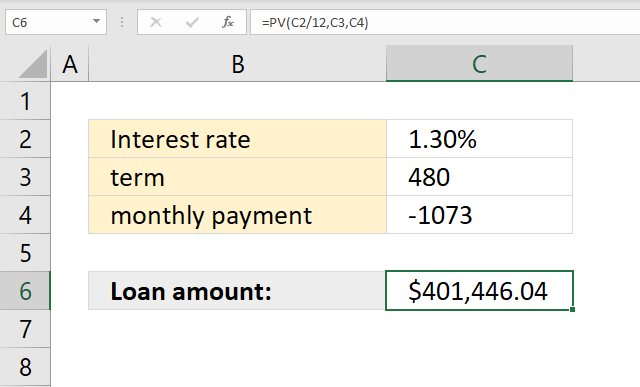The image above demonstrates a formula in cell C6 that calculates the loan amount based on an interest rate, the number of months needed to repay the loan and the monthly payment.

Formula in cell C6:

=PV(C2/12,C3,C4)

The PV function calculates the present value of a loan.

PV(rate, nper, pmt, [fv], [type])

## Example - PMT function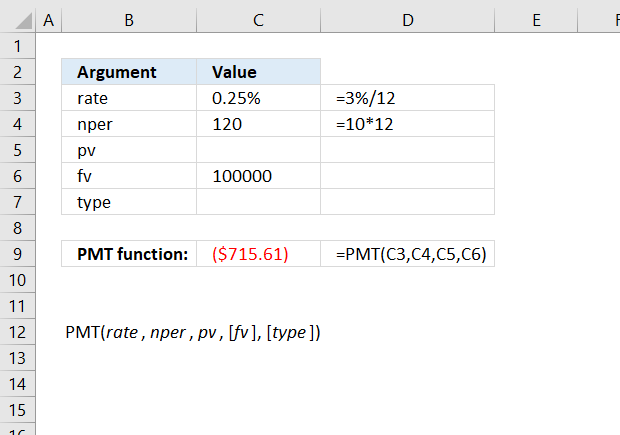The image above demonstrates how to calculate the monthly savings amount needed to reach 100 000 with a 3% interest rate and 10 years of savings.

Formula in cell C9:

=PMT(3%/12, 10*12, , 100000)